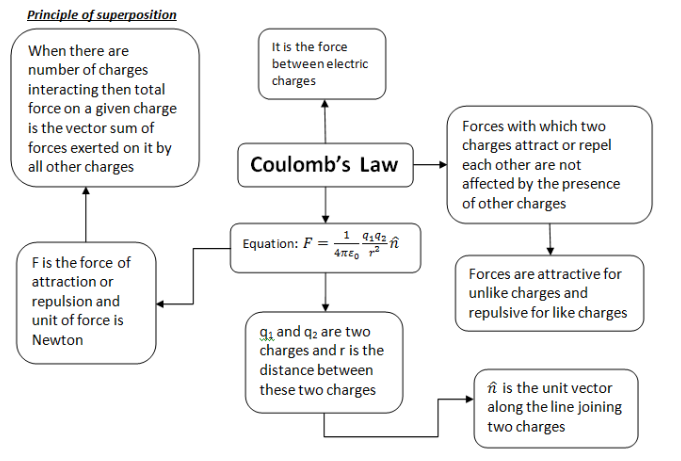According to the parallelogram law of addition. Experimentally it is verified that force on any charge due to a number of other charges is the vector sum of all the forces on that charge due to the other charges, taken one at a time. The individual forces are unaffected due to the presence of other charges. This is termed as the principle of superposition.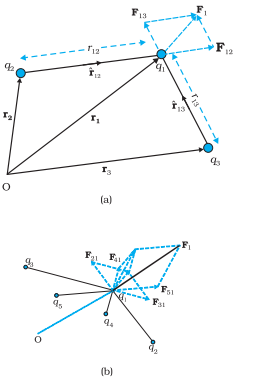Fig.2 A system of (a) three charges (b) multiple charges.

To better understand the concept, consider a system of three charges q1, q2, q3, as shown in Fig.2. The force on one charge, say q1, due to two other charges q2, q3 can, therefore, be obtained by performing a vector addition of the forces due to each one of these charges.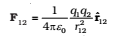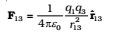Thus the total F1 on q1 due to the two charges q2 and q3 is given as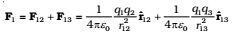The above calculation of force can be generalized to a system of charges more than three, as shown in fig.1(b)

The principle of superposition says that in a system of charges q1, q2, …, qn, the force on q1 due to q2 is the same as given by Coulomb’s law, i.e., it is unaffected by the presence of the other charges q3, q4, …, qn. The total force F1 on the charge q1, due to all other charges, is then given by the vector sum of the forces F12, F13,…,F1n: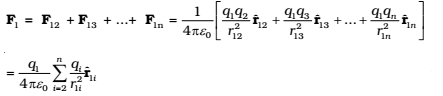The vector sum is obtained as usual by the parallelogram law of addition of vectors. All of the electrostatics is basically a consequence of Coulomb’s law and the superposition principle.